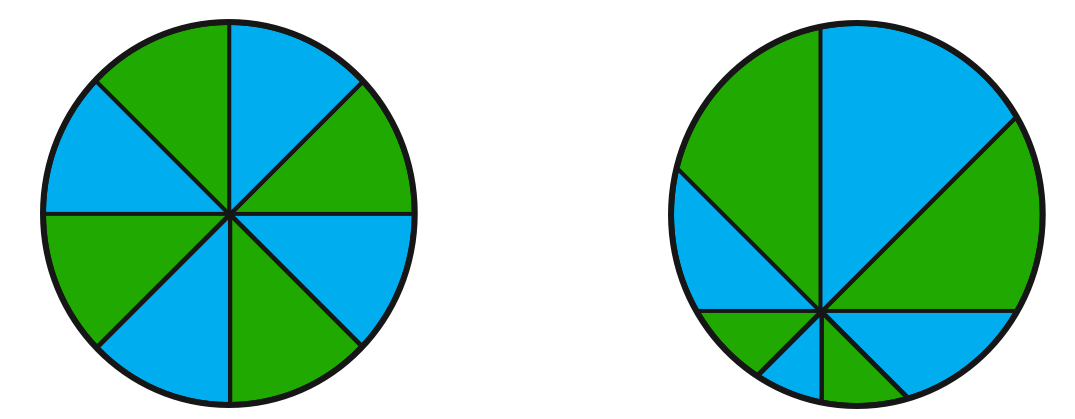# Let's Slice Some Pizza!

Geometry Level 1The above shows two circles. All of the acute angles between the lines in the circle are $45^\circ$.

In the left figure above, it is true that both the area of the blue region and the area of the green region are equal.

Can we say the same thing about the right figure above as well?

×